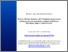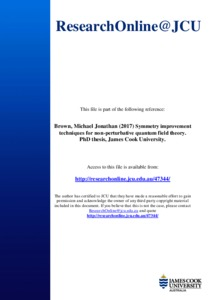# Symmetry improvement techniques for non-perturbative quantum field theory

Brown, Michael Jonathan (2017) Symmetry improvement techniques for non-perturbative quantum field theory. PhD thesis, James Cook University.Preview
PDF (Thesis)

## Abstract

Quantum field theory is the mathematical language of nature at the deepest levels currently known. Viewed in this light, much of the last century of theoretical physics can be seen as quantum field theory calculations performed in a variety of approximations. However, despite having a very successful standard model of physics and a phenomenally useful perturbation theory for extracting predictions from it, persistent problems remain. Many of these are due to the inability to calculate in practice what is calculable in principle. The difficulty of these problems comes from the importance of a large number of degrees of freedom, or a strong coupling between degrees of freedom, or both. Only in the case of very simple systems or systems close to thermal equilibrium can their properties be determined with confidence. The remaining cases require a resummation, or re-organisation, of perturbation theory. However, ad hoc resummations are problematic because perturbation series are asymptotic in nature and the re-organisation of the terms of an asymptotic series is mathematically questionable. Systematic resummation schemes are required to guarantee consistency with the original non-perturbative theory.

n-particle irreducible effective actions (nPIEAs; n=1,2,3,...) are a proven method of resummation which can be thought of as generalisations of mean field theory which are (a) elegant, (b) general, (c) in principle exact, and (d) have been promoted for their applicability to non-equilibrium situations. These properties make the nPIEAs compelling candidates for filling the gap between theory and experiment anywhere the problem is the inability to compute the behaviour of strongly interacting degrees of freedom or degrees of freedom which are out of thermal equilibrium. Unfortunately, nPIEAs are known to violate the symmetries of a field theory when they are truncated to a form that can be solved in practice. This can lead to qualitatively wrong physical predictions as an artefact of the method of calculation. If one takes the nPIEA predictions at face value one may reject a physically correct model on the basis of an incorrect prediction. Therefore it is critical to gain a better understanding of the symmetry problem in order for nPIEAs to be useful in the future.

This is where this thesis aims to make a contribution. This thesis examines the theory of global symmetries in the nPIEA formalism for quantum field theory with the goal of improving the symmetry properties of solutions obtained from practical approximation schemes. Following pedagogical reviews of quantum field theory and nPIEAs, symmetries are introduced by considering a scalar (ϕ²)² field theory with O(N) symmetry and spontaneous symmetry breaking. The symmetries are embodied by Ward identities which relate the various correlation functions of the theory. When truncated, the nPIEAs for n>1 violate these identities with phenomenological consequences which are reviewed. The symmetry improvement method (SI) of Pilaftsis and Teresi (Nuclear Physics B, 874(2):594-619, 2013) addresses this by imposing the 1PI Ward identities directly onto the solutions of nPI equations of motion through the use of Lagrange multipliers. In novel work, SI is extended to the 3PIEA. The SI-3PIEA is then renormalised in the Hartree-Fock and two loop truncations in four dimensions and in the three loop truncation in three dimensions. The Hartree-Fock equations of motion are solved and compared to the unimproved and SI-2PI Hartree-Fock solutions. In both the SI-2PI and SI-3PI methods Goldstone's theorem is satisfied. However, the SI-2PI solution correctly predicts that the phase transition is second order while the SI-3PI solution predicts a weak (weaker than the unimproved 2PIEA) first order transition. Further checks of the formalism are performed: it is shown that the SI-3PIEA obeys the Coleman-Mermin-Wagner theorem and is consistent with unitarity to O (ℏ), but only if the full three loop truncation is kept.

Following this a novel method of soft symmetry improvement (SSI) is introduced. SSI differs from SI in that the Ward identities are imposed softly in the sense of least squared error. A new stiffness parameter controls the strength of the constraint. The motivation for this method is that the singular nature of the constraint in SI leads to pathological behaviour such as the non-existence of solutions in certain truncations and the breakdown of the theory out of equilibrium. In order to regulate the IR behaviour, a box of finite volume is used and the limit of infinite volume taken carefully. Three limiting regimes are found. Two are equivalent to the unimproved 2PIEA and SI-2PIEA respectively. The third is a novel limit which has pathological behaviour including a strongly first order phase transition and a regime where solutions cease to exist for a range of temperatures below the critical temperature. As the stiffness increases this range increases and at a finite value of the stiffness the solution ceases to exist even at zero temperature. This loss of solution is confirmed both analytically and numerically.

Finally, the linear response of a system in equilibrium subject to external perturbations is studied for the first time in the SI-2PIEA formalism. It is shown that, beyond equilibrium, the scheme is inconsistent in that the equations of motion are generically over-constrained. Thus, the SI-2PIEA predicts qualitatively incorrect physics out of equilibrium. This result can be understood as a result of the decoupling of the propagator fluctuation from the field fluctuation in the 2PIEA formalism and the failure of higher order Ward identities. This argument generalises so that, as a result, SI-nPIEA are invalid out of equilibrium, at least within the linear response approximation in generic truncations.

Item ID: 47344 Thesis (PhD) quantum field theory; symmetry; symmetry improvement; effective action; non-perturbative; non-equilibrium For this thesis, Michael Brown received the Dean's Award for Excellence 2018.See Related URLs for a link to the source distribution including all code.Publications arising from this thesis are available from the Related URLs field. The publications are:Brown, Michael J., and Whittingham, Ian B. (2015) Symmetry improvement of 3PI effective actions for O(N) scalar field theory. Physical Review D (Particles, Fields, Gravitation and Cosmology), 91 (8). pp. 1-27.Brown, Michael, and Whittingham, Ian (2015) Two-particle irreducible effective actions versus resummation: analytic properties and self-consistency. Nuclear Physics B, 900. pp. 477-500.Brown, Michael, Whittingham, Ian B., and Kosov, Daniel S. (2016) Linear response theory for symmetry improved two particle irreducible effective actions. Physical Review D, 93 (10). pp. 1-13.Brown, Michael J., and Whittingham, Ian B. (2017) Soft symmetry improvement of two particle irreducible effective actions. Physical Review D, 95 (2). pp. 1-18. 16 Aug 2017 01:42 02 PHYSICAL SCIENCES > 0206 Quantum Physics > 020602 Field Theory and String Theory @ 34%01 MATHEMATICAL SCIENCES > 0105 Mathematical Physics > 010505 Mathematical Aspects of Quantum and Conformal Field Theory, Quantum Gravity and String Theory @ 33%02 PHYSICAL SCIENCES > 0203 Classical Physics > 020304 Thermodynamics and Statistical Physics @ 33% 97 EXPANDING KNOWLEDGE > 970102 Expanding Knowledge in the Physical Sciences @ 70%97 EXPANDING KNOWLEDGE > 970101 Expanding Knowledge in the Mathematical Sciences @ 30% Total: 162 Last 12 Months: 10 More Statistics

Actions (Repository Staff Only)Item Control Page